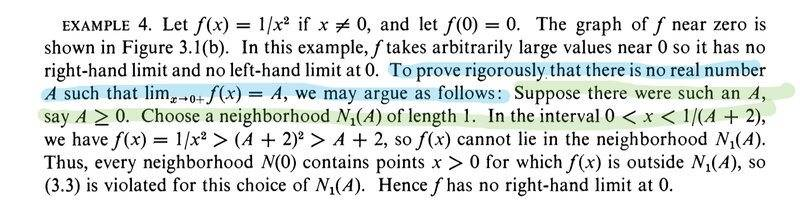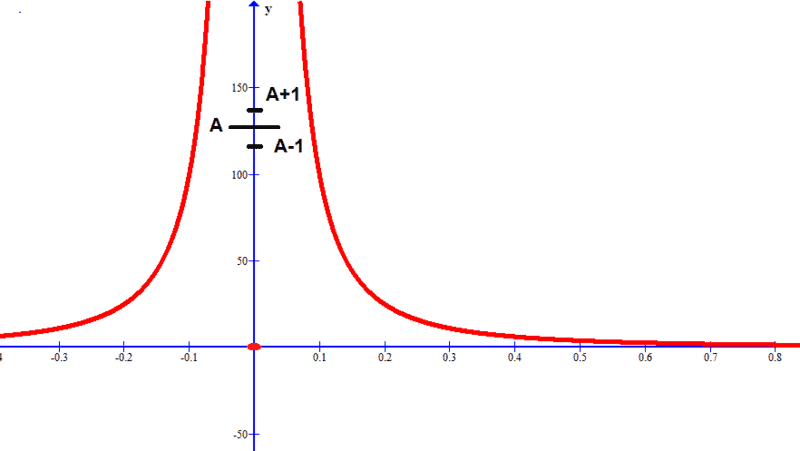# Proof in Apostol Calculus Pg. 130 Doubt (proving that 1/(x^2) does not have a limit at 0)

In Apostol’s Calculus (Pg. 130) they are proving that 1/(x^2) does not have a limit at 0. In the proof, I am unable to understand how they conclude from the fact that the value of f(x) when 0 < x < 1/(A+2) is greater than (A+2)^2 which is greater than A+2 that every neighborhood N(0) contains points
x > 0 for which f(x) is outside N1(A). I don’t get how they generalized the specific statement to all neighborhood of N(0). Thank you!fresh_42
Mentor
The proof is correct. Draw a picture:There is no value ##f(x)## in ##N_1(A)## and you can do this for any ##A##, so ##\lim_{x \to 0^+}f(x) \neq A##. ##A=0## is also impossible, since ##0## isn't a limit point.

Delta2
Homework Helper
Gold Member
This is a subtle point and requires to have perfect understanding of the exist and for all quantifiers in the epsilon-delta definition of the limit. More specifically if one wants to prove that A is NOT a limit as x->0 then he has to prove that
$$\exists \epsilon>0 : \forall \delta>0 ,\exists x:0<|x-0|<\delta\Rightarrow |f(x)-A|>\epsilon$$

The epsilon is ##\epsilon=1## and the delta is any positive number because for any such delta there exists ##x<min(\delta,\frac{1}{A+2})## for which ##0<|x-0|<\delta## and ##|f(x)-A|>\epsilon=1##. We can choose any ##x<min(\delta,\frac{1}{A+2})## but for the proof we require to exist at least one such x (for every different delta).

So we don't actually have to prove that all the points of every neighborhood ##N(0)## are such that ##|f(x)-A)|>1##, but just that for every neighborhood## N(0)## there exists at least one point ##x ## such that ##|f(x)-A|>1##.

Last edited:
PeroK
Homework Helper
Gold Member
2020 Award
In Apostol’s Calculus (Pg. 130) they are proving that 1/(x^2) does not have a limit at 0. In the proof, I am unable to understand how they conclude from the fact that the value of f(x) when 0 < x < 1/(A+2) is greater than (A+2)^2 which is greater than A+2 that every neighborhood N(0) contains points
x > 0 for which f(x) is outside N1(A). I don’t get how they generalized the specific statement to all neighborhood of N(0). Thank you!

View attachment 288821
This seems like a slightly odd argument to me. The right-hand limit is clearly ##+\infty##, which can be proved quite easily. Then, you must have a general theorem that a limit cannot be both a finite number ##A## and ##+\infty##. That can be done once for all cases.

For the same effort, you could have a theorem rather than a statement about a single function at a single point.

pasmith
Homework Helper
In Apostol’s Calculus (Pg. 130) they are proving that 1/(x^2) does not have a limit at 0. In the proof, I am unable to understand how they conclude from the fact that the value of f(x) when 0 < x < 1/(A+2) is greater than (A+2)^2 which is greater than A+2 that every neighborhood N(0) contains points
x > 0 for which f(x) is outside N1(A). I don’t get how they generalized the specific statement to all neighborhood of N(0). Thank you!

View attachment 288821

The intersection of $N(0)$ and $(0, 1/(A + 2))$ is $(0, x_0)$ where $$x_0 = \min\{ \sup N(0), 1/(A + 2)\} > 0$$ since both $\sup N(0)$ and $1/(A + 2)$ are strictly positive. This intersection is never empty.

Last edited:
WWGD